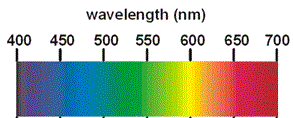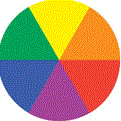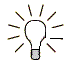# Colorimetry (Colourimetry) Chemistry Tutorial

## Key Concepts

• Colorimetry (colourimetry) is also referred to as colorimetric analysis (colourimetric analysis).
• Colorimetry (colourimetry) is an analytical technique used by Chemists to determine the concentration of a coloured solution.
• A solution is coloured because it absorbs different amounts of the different wavelengths that make up "white light".
• A colorimeter (colourimeter) is an instrument used to measure the absorbance of light by a coloured solution.(1)
• The wavelength chosen for colorimetric analysis is that which gives maximum absorption for the coloured solution.

We can use a "colour wheel" to determine the colour of light to use.(2)

• The intensity of the colour of a solution is related to the concentration of the solution.

The more concentrated the solution is, the more intense the colour will be.

• To determine the concentration of a solution using colorimetry:

(1) Determine the wavelength (colour) of light to use for the colorimetric analysis.

(2) Prepare a set of standard solutions of known concentration.

(3) Measure the absorbance of each standard solution using the colorimeter.

(4) Plot the absorbance vs concentration for each standard solution on a graph.(3)

(5) Draw the line of best fit through the data points. This is the calibration curve.

(6) Measure the absorbance of the solution of unknown concentration using the colorimeter.

(7) Use the calibration curve to determine the concentration of this solution.

No ads = no money for us = no free stuff for you!

## Colour and Absorption of Light

Many solutions can be coloured.
You may already be familiar with some coloured aqueous solutions, for example:

• copper sulfate dissolved in water produces a blue solution (CuSO4(aq))
• potassium permanganate dissolved in water produces a purple solution (KMnO4(aq))
• iron(III) thiocyanate dissolved in water produces a red solution (FeSCN2+(aq))
• bromine water is a red-brown solution (Br2(aq))
• iodine dissolved in water is a yellow-brown solution
• the complex formed between starch and iodine is a deep blue colour
• acid-base indicator solutions come in a wide range of colours

The colour we see is the colour of the light that is transmitted through the solution.
"White light" is part of the electromagnetic spectrum and is made up of all the colours of the rainbow; red, orange, yellow, green, blue, indigo and violet (ROYGBIV).If we shine white light through an aqueous solution of copper sulfate, the solution appears to be blue because it is transmitting the blue wavelengths of white light and absorbing other wavelengths such as red so that very little of these other wavelengths are transmitted through the solution to your eye.

 → white light enters → solution → light transmitted to your eye → → violet → CuSO4(aq) → indigo → → blue → → green → → yellow → → orange → → red →

The colour of the solution is due to the wavelengths of light that are not absorbed as "white light" passes through the solution.

Now, if we dilute the original copper sulfate solution by adding more water, the blue colour becomes less intense, it is a lighter blue colour because the solution is not absorbing as much of the other wavelengths as before:

concentrated solution
(0.50 mol L-1)
dilute solution
(0.10 mol L-1)
 white light → solution → light transmitted → violet → CuSO4(aq) → indigo → → blue → → green → → yellow → → orange → → red →

 white light → solution → transmitted light → violet → CuSO4(aq) → indigo → → blue → → green → → yellow → → orange → → red →

If we were given an aqueous solution of copper sulfate of unknown concentration, solution (A), we might be able to take a guess at its concentration by looking at its colour:

solution (A)

The "blueness" of solution (A) seems to lie about half-way between that of the concentrated (0.50 mol L-1) solution and the dilute (0.10 mol L-1) solutions above, so we might guess that the concentration of solution (A) lies about halfway between these two solutions: (0.50 + 0.10) ÷ 2 = 0.30 mol L-1.

Guessing the concentration of the solution in this way gives us a kind of "ball park" value for the concentration, but it is unlikely to result in us knowing the concentration of the solution accurately or precisely.
But there might be a better way.
We have already noted that there is a relationship between the intensity of the colour of solutions and their concentration.
And we have seen that a concentrated blue solution will absorb more of the "non-blue" wavelengths of light than a more dilute solution, and we have a machine, a colorimeter (colourimeter), that can measure the amount of absorbance for us!

Do you know this?

Play the game now!

## Colorimeters (Colourimeters)

Colorimetry (colourimetry) is the name of the method we use to determine the concentration of a substance by measuring the relative absorption of light with respect to known concentrations of the substance.

A colorimeter (colourimeter) is literally a meter to measure colour. It is an instrument that measures the absorption of light of a specific wavelength (λ) by a solution.(4)

Therefore, we need to choose a wavelength of light to shine through our solution.
The solution must be able to absorb measurable amounts of this wavelength.
Different concentrations of the solution must absorb different amounts of this wavelength, and this difference must be significant (that is, much larger than the error inherent in the measurment).

If a solution is blue, like CuSO4(aq), we would not use a wavelength corresponding to blue light because very little of this is absorbed (most is being transmitted).
Similarly, lots of the green and indigo wavelengths are not absorbed but are transmitted.
Therefore we would choose to shine light from the "red-orange-yellow" part of the spectrum at this solution because these are the wavelengths that are being absorbed.

We can use a "colour wheel" to decide which is most likely to be the best colour of light to shine on our solution:If our solution is blue, we choose a light colour that is complementary to blue, that is, we choose the colour that is opposite this colour in the colour wheel, in this case we choose an orange light to shine on our blue solution.

Colour of
Solution
Colour of Light to
Use in Colorimeter
yellow purple
orange blue
red green
purple yellow
blue orange
green red

Now, it may be possible to select an appropriate wavelength for the light our colorimeter uses, but it is quite likely that our colorimeter will use coloured filters in order to "filter out" (absorb) the wavelengths of light that we do not want from "white light".
In this case, if we want to determine the amount of orange light absorbed by our blue solutions, we would select an orange filter for our colorimeter.

A simplified schematic diagram of using an orange filter in a colorimeter to measure the concentration of a blue copper sulfate solution is shown below:

 "white" light source slit orangefilter CuSO4(aq) detector / recorder→ → → → detector →

Light from the "white light source" passes through a slit then a filter to select the most appropriate wavelength of light. In this case, the orange filter absorbs all other wavelengths and allows the orange wavelengths to be transmitted through the filter.
Some of this "orange light" is then absorbed by the "blue solution" which is contained in a glass receptacle.(5)
The intensity of the "orange light" has decreased after it passes through the "blue solution" because the "blue solution" has absorbed a significant amount of "orange".

The amount of light absorbed is measured and recorded as the "absorbance".
The more concentrated the solution the greater the "absorbance" will be.

## Determining the Concentration of a Solution Using A Colorimeter (Colourimeter)

A colorimeter (colourimeter) measures the absorption of light of a specific wavelength (λ) by a solution.
The colorimeter records this as the absorbance.

In order to determine the concentration of a solution, we will need to obtain the absorbances for a range of different concentrations of the same solution using the same wavelength of light (same filter).
We use the absorbance of each of these solutions of known concentration to draw a calibration curve.
We can then measure the absorbance of our solution of unknown concentration and use the calibration curve to determine its concentration.

If, for example, we have an aqueous solution of copper sulfate, CuSO4(aq), and we want to determine its concentration colorimetrically, we would first prepare a stock solution of aqueous copper sulfate.
We then use this stock solution to prepare a number of dilute copper sulfate solutions, calculating the concentration of each solution.
Note that we should visually check the colour of our unknown solution against the colour of each of the prepared solutions in order to ensure that the colour of our solution lies between the colour of the most concentrated and most dilute solution we have prepared.
Next we use the colorimeter (colourimeter) to measure the absorbance of each of these solutions of known concentration.
The results of this experiment might look like those in the table below:

[CuSO4(aq)]
(mol L-1)
Absorbance
(λ = 635 nm)
0.00 0.000
0.10 0.280
0.20 0.560
0.30 0.830
0.40 1.110
0.50 1.390

Next we plot these results on a graph and draw a "line of best fit" through the data points:

 absorbance Calibration Curve[CuSO4(aq)] / mol L-1

Now we measure the absorbance of our aqueous solution of copper sulfate of unknown concentration.

Example: Absorbance of unknown solution = 0.950

We plot the point (x) for this unknown solution on our calibration curve:

 absorbance Calibration Curve[CuSO4(aq)] / mol L-1

Reading off the graph we see that an absorbance of 0.950 corresponds to a concentration of 0.34 mol L-1

Alternatively, if we know the mathematical equation for the straight line of the calibration curve, we can use that to determine the concentration of our unknown solution.
On the calibration graph we were given the expression:

Abs = 2.78 × [CuSO4(aq)]

which is the mathematical equation for the calibration curve.
Substitute the value for the absorbance of our unknown solution into the equation:

0.950 = 2.78 × [CuSO4(aq)]

Divide both sides of the equation by 2.78:

(0.950 ÷ 2.78) = (2.78 ÷ 2.78) × [CuSO4(aq)]

Solve the equation to find the concentration of our unknown solution:

0.3417 mol L-1 = [CuSO4(aq)]

However, we are only justified in using 2 significant figures to express the concentration of our unknown solution, that is:

[CuSO4(aq)] = 0.34 mol L-1

In summary, to determine the concentration of a coloured solution using colorimetry:

1. Determine the wavelength (colour) of light to use for the colorimetric analysis.
2. Prepare a set of standard solutions of known concentration.
3. Measure the absorbance of each standard solution using the colorimeter.
4. Plot the absorbance vs concentration for each standard solution on a graph.
5. Draw the line of best fit through the data points. This is the calibration curve.
6. Measure the absorbance of the solution of unknown concentration using the colorimeter.
7. Use the calibration curve to determine the concentration of this solution.

Do you understand this?

Take the test now!

## Worked Example: Using Colorimetry to Determine the Concentration of A Solution

The Problem: Chris the Chemist needs to determine the concentration of a deep blue copper sulfate solution.
First Chris transfers 25.00 mL of the solution by pipette into a 100.00 mL volumetric flask and makes the solution up to the mark with distilled water.
Next, Chris prepares copper sulfate solutions of known concentration and measures their absorbance in order to establish a calibration curve as shown below:

 absorbance Calibration Curve[CuSO4(aq)] / mol L-1

Then Chris measures the absorbance of the diluted solution and finds that it is 0.506
Determine the concentration of the original copper sulfate solution.

Solving the Problem using the StoPGoPS model for problem solving:

STOP! State the question. What is the question asking you to do?

Determine the concentration of the original copper sulfate solution.

[CuSO4(aq)] = ? mol L-1

PAUSE! Plan.

What information (data) have you been given?

Calibration curve as shown above.

Absorbance of diluted unknown solution = 0.506

100.00 mL of dilute solution contains 25.00 mL of original solution

What is the relationship between the data you have been given and what the question is asking you to do?

Step 1: Plot the absorbance for the diluted solution on the calibration curve to determine its concentration.

Step 2: Determine the concentration of the undiluted original solution:

ciVi = cfVf

ci = [CuSO4(aq)(original)] = ? mol L-1

Vi = volume of CuSO4(aq)(original) = 25.00 mL

cf = [CuSO4(aq)(diluted)] = ? mol L-1 (from calibration curve)

Vf = volume of CuSO4(aq)(diluted) = 100.00 mL

GO! Go with the Plan.

Step 1: Plot the absorbance for the diluted solution on the calibration curve to determine its concentration.

 absorbance Calibration Curve[CuSO4(aq)] / mol L-1

[CuSO4(aq)(diluted)] = 0.18 mol L-1

Step 2: Determine the concentration of the undiluted original solution:

ciVi = cfVf

ci = [CuSO4(aq)(original)] = ? mol L-1

Vi = volume of CuSO4(aq)(original) = 25.00 mL = 25.00 mL ÷ 1000 mL/L = 0.02500 L

cf = [CuSO4(aq)(diluted)] = 0.18 mol L-1 (from calibration curve)

Vf = volume of CuSO4(aq)(diluted) = 100.00 mL = 100.00 mL ÷ 1000 mL/L = 0.10000 L

ci × 0.02500 L = 0.18 mol L-1 × 0.10000 L

ci × 0.02500 L = 0.018 mol

ci × 0.02500 L ÷ 0.02500 L = 0.018 mol ÷ 0.02500 L

ci = 0.72 mol L-1 (only justified in 2 significant figures)

Yes, we have determined the concentration of the original, undiluted, copper sulfate solution.

Is your solution to the question reasonable?

Consider the absorbance of the diluted solution (0.506). Looking at the graph we see that this lies just below the absorbance for 0.20 M CuSO4(aq) which is 0.560 so we expect the concentration of our dilute solution to be a bit less than 0.20 M which it is (0.18 M)
We can also use the equation for the calibration curve given on the graph to calculate the concentration:
Abs = 2.81 × [CuSO4(aq)]
0.506 = 2.81 × [CuSO4(aq)]
0.506 ÷ 2.81 = [CuSO4(aq)] = 0.18 M
So we are confident that the concentration of the dilute solution was 0.18 M

Next, compare the relative concentrations of the diluted and undiluted (original) solutions.
The original (undiluted) solution must have a greater concentration than the diluted solution.
[CuSO4(aq)(diluted)] = 0.18 M
[CuSO4(aq)(undiluted)] = 0.72 M
[CuSO4(aq)(undiluted)] > [CuSO4(aq)(diluted)] since 0.72 M > 0.18 M
So we are reasonably confident that our answer is correct.

STOP! State the solution. What is the concentration of the original copper sulfate solution?

[CuSO4(aq)(original)] = 0.72 mol L-1

Can you apply this?

Join AUS-e-TUTE!

Take the exam now!

Footnotes:

(1) There are different types of colorimeter.
When white light is used with a simple colorimeter to compare the colour of light by eye, we refer to this as visual colorimetry.
When the human eye is replaced by a photoelectric cell the instrument is referred to as a photoelectric colorimeter.
A filter photometer refers to using coloured filters as described in this tutorial in the photoelectric colorimeter. The filters may be made of coloured glass or gelatin.
A UV-visible spectrophotometer can be used and then we refer to this as spectrophotometric analysis. You can find out more about this in our UV-Visible Spectroscopy tutorial.

(2) The "colour wheel" gives us an indication of which coloured "filter" we should use.
Instead of a "colour wheel" you might be given a list of complementary colours, again, this is just giving you an indication of which "filter" to use in the colorimeter:

Wavelength (nm) Colour Transmitted Complementary Colour
400 - 435 violet yellowish-green
435 - 480 blue yellow
480 - 490 greenish-blue orange
490 - 500 bluish-green red
500 - 560 green purple
560 - 580 yellowish-green violet
580 - 595 yellow blue
595 - 610 orange greenish-blue
610 - 750 red bluish-green

A better way to choose a filter is to first construct an absorption curve using a spectrophotometer and compare this to the set of filters available.
For this introductory tutorial however, we are more interested in the general principles and application of colorimetry rather than in the detailed specifics so we will use a "colour wheel".

(3) There are other methods for using colorimetry to determine the concentration of a solution, however, for this introductory tutorial we will use the simplest approach, that is, we will draw a calibration curve.

(4) Well ... more or less a specific wavelength if you are using coloured filters. That is, the filters restrict the wavelengths to a narrow range.

(5) The type of vessel used in your colorimetry experiment depends on the colorimeter you use.
You might use a glass tube known as a Nesslar tube. A Nesslar tube looks like a test tube except that it has a flat bottom.
Or you might use a cuvette (curvet) instead. A cuvette looks less like a test tube because it is rectangular instead of circular in cross-section and has a flat bottom.
The important thing is that the receptacle you use to hold your solutions is colourless and has a uniform cross-section.